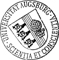## Uniform Convergence of Local Multigrid Methods for the Time-harmonic Maxwell Equation

• For the efficient numerical solution of indefinite linear systems arising from curl conforming edge element approximations of the time-harmonic Maxwell equation, we consider local multigrid methods (LMM) on adaptively refined meshes. The edge element discretization is done by the lowest order edge elements of Nedelec's first family. The LMM features local hybrid Hiptmair smoothers of Jacobi and Gauss-Seidel type which are performed only on basis functions associated with newly created edges/nodal points or those edges/nodal points where the support of the corresponding basis function has changed during the refinement process. The adaptive mesh refinement is based on Dörfler marking for residual-type a posteriori error estimators and the newest vertex bisection strategy. Using the abstract Schwarz theory of multilevel iterative schemes, quasi-optimal convergence of the LMM is shown, i.e., the convergence rates are independent of mesh sizes and mesh levels provided the coarsest mesh isFor the efficient numerical solution of indefinite linear systems arising from curl conforming edge element approximations of the time-harmonic Maxwell equation, we consider local multigrid methods (LMM) on adaptively refined meshes. The edge element discretization is done by the lowest order edge elements of Nedelec's first family. The LMM features local hybrid Hiptmair smoothers of Jacobi and Gauss-Seidel type which are performed only on basis functions associated with newly created edges/nodal points or those edges/nodal points where the support of the corresponding basis function has changed during the refinement process. The adaptive mesh refinement is based on Dörfler marking for residual-type a posteriori error estimators and the newest vertex bisection strategy. Using the abstract Schwarz theory of multilevel iterative schemes, quasi-optimal convergence of the LMM is shown, i.e., the convergence rates are independent of mesh sizes and mesh levels provided the coarsest mesh is chosen sufficiently fine. The theoretical findings are illustrated by the results of some numerical examples.• Dokument_1.pdfAuthor: Huangxin Chen, Ronald H. W. HoppeGND, Xuejun Xu urn:nbn:de:bvb:384-opus4-11943 https://opus.bibliothek.uni-augsburg.de/opus4/1472 Preprints des Instituts für Mathematik der Universität Augsburg (2011-01) Preprint English Universität Augsburg Chinese Academy of Sciences, University of Houston 2011/01/04 time-harmonic Maxwell equation; local multigrid methods; Hiptmair smoothers; adaptive edge element methods; optimality Maxwellsche Gleichungen; Mehrgitterverfahren; Numerisches Verfahren Mathematisch-Naturwissenschaftlich-Technische Fakultät Mathematisch-Naturwissenschaftlich-Technische Fakultät / Institut für Mathematik Mathematisch-Naturwissenschaftlich-Technische Fakultät / Institut für Mathematik / Lehrstuhl für Numerische Mathematik 5 Naturwissenschaften und Mathematik / 51 Mathematik / 510 MathematikDeutsches Urheberrecht mit Print on Demand